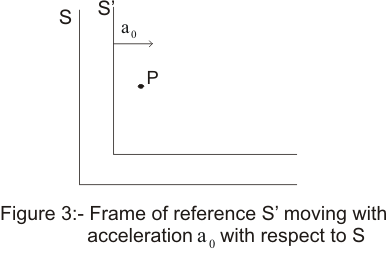# Inertial frame of reference|Pseudo forces

## (8) Inertial frame of Reference

• Inertial frame of references are those frames of reference in which newton's first and second law of motion is always hold true
• A frame of reference in which Newton's law are not valid is called non-inertial frame of reference
• In an inertial frame if a body is not acted by external force ,it continues to be in state of rest or uniform translatory motion.Thus in an inertial frame if the body is not acted upon by an external force then acceleration would be zero
a=(d2r/dt2)=0
• If a frame is inertial frame ,then all those frames which are moving with constant velocity relative to the previous frame are also inertial frames
• Inertial frame of reference are necessary unaccelerated frames because if the frame is accelerated the particle moving with uniform velocity will appear

### (9) Fictitious ( or Pseudo ) Forces

• We already know about Non-inertial frame of reference .All the accelrated and rotatig frame of reference are non-inertial frame of refrence
• Consider an interial frame of reference S and let S' be any other frame moving with accleration w.r.t to frame S as shown in the below figure• Now if no external forces are acting on particle P .Then its acceleration would be zero in Frame S but in frame S',an observer will find an acceleration -a0 acting on the particle.
• The observer force on particle P of mass m in Frame S' is -ma0
• But in reality no such force is acting on the particle and particle appears to be accelerated in this non-inertial frame of reference.Such one force is known as Pseudo or Fictitious Force. Hence Pseudo Force on particle is
FP=-ma0
• Now if we apply Fi on the particle and ai is the observed acceleration of particle in S frame(Inertial frame) The according to Newton's law
Fi=mai
• For calculating net force in accelerated frame consider both the frames S and S' coincide at time t=0 .After time t let ri and rn be the position vector of the particle in frame S and S' respectively
The relation between ri and rn is
ri =rn +(1/2)a0t2 Where a0 is the acceleration of frame S' wrt frame S
Differentiating the equation w.r.t time twice
ai=an + a0
or mai-ma0=man
=> F0 + FP=FN

This equation gives observed force in accelerated frame of reference